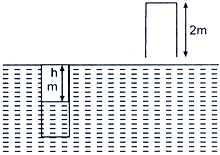# A 2m long tube closed at one end is lowered vertically into water until the closed end is flush with the water surface. See figure below. Calculate the water level height in the tube. (Barometric pressure – 1 atm = 10 m of hydrostatic water head, Temperature = 25oC, Density of water = 1.00 g/ml. Neglect water vapour pressure).(A)    1.01 m        (B)    0.29 m        (C)    1.71 m        (D)    0.92 m

9 years ago

(B)

Sol.   PV = nRT

V =  . T   Þ V = KT

\      log P = log T + log K

Linear dependence with positive slope

At the Y-intercept P/t®0 and P®0 inplying P®0, under such conditions all gases show ideal behavior.

5 years ago
Questions is based on Boyle’s law
before the tube is lowered vertically into water, P * V = 1 atm * (2m * base area of tube) (as volume = base area * height).
after tube is lowered into water, the pressure experienced by gas inside the tube is

Now, as 1atm is equivalent to 10 m of height of water we can say

in atm
i.e. Pgas = 1.2 – (2 – h) / 10 atm
and volume of gas column = base area * h m

Thus, applying boyle’s law,
1 * 2 * base area = {1.2 – (2 -h)/10} * base area * h
solving for h, we get h = 1.708 m